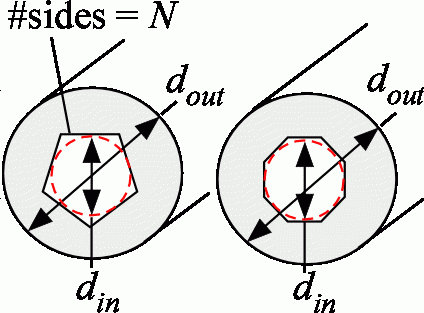Contents - Index

SF_28Function SF_28(d_in, d_out, L, N) returns the shape factor for conduction between an N-sided polygon shaped hole that is centered inside a circular extrusion.

The calling protocol is:

S= SF_28(d_in, d_out, L, N)

Inputs:

d_in = diameter of the circle inscribed within the polygon [m or ft]

d_out = diameter of the extrusion [m or ft]

L = length of the extrusion [m or ft]

N = number of sides [-]

Outputs:

S shape factor [m or ft]

Rohsenow, W.M, J. P. Hartnett, and Y. I. Cho, Handbook of Heat Transfer, 3rd Edition, McGraw Hill, (1998).

Example:

\$UnitSystem SI Mass J K Pa

d_in=0.1 [m]

d_out = 0.2 [m]

L = 1 [m]

N=4 [-]

S = sf_28(d_in,d_out,L,N)

{Solution:

S=8.971 [m]}